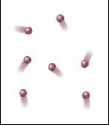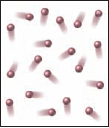×
Get Full Access to Introductory Chemistry - 5 Edition - Chapter 11 - Problem 123p
Get Full Access to Introductory Chemistry - 5 Edition - Chapter 11 - Problem 123p

×

# Which gas sample has the greatest pressure? Assume they are all at the same temperatureISBN: 9780321910295 34

## Solution for problem 123P Chapter 11

Introductory Chemistry | 5th Edition

• Textbook Solutions
• 2901 Step-by-step solutions solved by professors and subject experts
• Get 24/7 help from StudySoup virtual teaching assistantsIntroductory Chemistry | 5th Edition

4 5 1 392 Reviews
20
4
Problem 123P

PROBLEM 123P

Which gas sample has the greatest pressure? Assume they are all at the same temperature. Explain.

(a)(b)(c)Step-by-Step Solution:

Solution 123P

Here, we are going to identify the gas sample having greatest pressure.

Step 1:

At constant temperature and volume, the pressure(p) of a gas is directly proportional to the number of moles(n).

Thus,

p ∝ n

Step 2 of 3

Step 3 of 3

##### ISBN: 9780321910295

This textbook survival guide was created for the textbook: Introductory Chemistry, edition: 5. Introductory Chemistry was written by and is associated to the ISBN: 9780321910295. The full step-by-step solution to problem: 123P from chapter: 11 was answered by , our top Chemistry solution expert on 05/06/17, 06:45PM. The answer to “Which gas sample has the greatest pressure? Assume they are all at the same temperature. Explain.(a) (b) (c)” is broken down into a number of easy to follow steps, and 18 words. This full solution covers the following key subjects: assume, explain, gas, greatest, pressure. This expansive textbook survival guide covers 19 chapters, and 2046 solutions. Since the solution to 123P from 11 chapter was answered, more than 2133 students have viewed the full step-by-step answer.

Unlock Textbook Solution